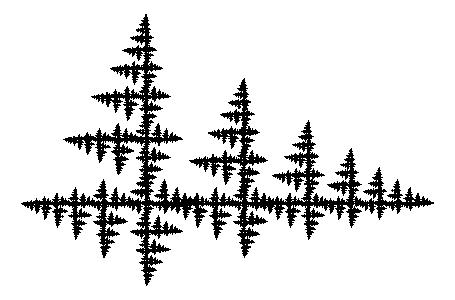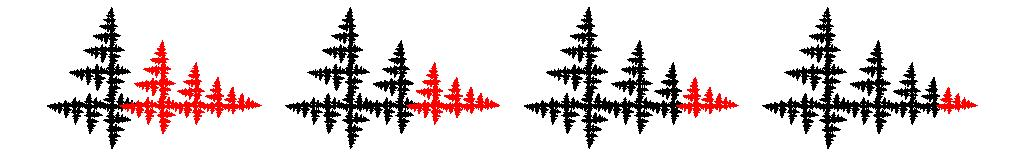# Iterated Function Systemsfigure 1: a fractal defined by a contraction and a rotation followed by a contraction

Except for a few of the examples on this site, all of the fractals produced by elmfract are attractors of iterated function systems. The functions of the iterated function system are two or more geometrical transformations in two- or three-dimensional space. The resulting fractal itself is called an attractor because when the functions are applied to a set of points in space repeatedly, the resulting points are attracted to the fractal. Mathematicians use the term iterative to indicate a procedure that is applied repeatedly.

In this description I will first discuss some of the transformations I am using in elmfract, and then show how attractors attract. All the elmfract transformations contain at least a contraction to a point, most contain other transformations as well, such as reflections or rotations. As an example, I will discuss a two-dimensional fractal that is defined by two transformations, a contraction by a factor of 0.66, and a rotation over 90 degrees counter clockwise followed by a contraction by the same factor of 0.66. The resulting fractal is shown here in figure 1.

## Geometrical Transformations: a Contraction

figure 2: animation of a contraction transformation

This contraction scales the fractal by a factor of 0.66 in such a way that the rightmost point remains in place. As a result the fractal contains an infinite number of complete scaled copies of itself.

The animation in figure 2 shows the contraction with different colors for the different scaled versions. Figure 3 shows in red the scaled versions of the fractal that are present after one, two, three, and four times applying of the contraction.figure 3: application of the contraction transformation on the whole fractal one, two, three, and four times

## Geometrical Transformations: a Contracting Rotation

figure 4: animation of a contracting rotation transformation

This contracting rotation rotates the fractal over 90 degrees and then scales it by a factor of 0.66. Due to this transformation the fractal contains an inifite number of rotated and scaled copies of itself.

The animation of figure 4 shows that the rotation point is left from the center of the fractal. Figure 5 shows the effect of applying the contracting rotation multiple times on the fractal as a whole in blue.figure 5: application of the contracting rotation transformation on the whole fractal one, two, three, and four times

## Combinations of transformationsfigure 6: left: first a contraction, then a contracting rotation; right: first a contracting rotation, then a contraction

Combinations of the transformations reveal other scaled copies of the fractal that are present. In figure 6 two combinations of the two contributing transformations are shown in purple. This fractal really consists of an infinite number of smaller copies of itself.

## The Attraction of the Attractor

figure 7: a contraction working on a red, and a contracting rotation working on a blue block of points

Now let's get back to the issue of the attractor. As an example we take a slight variation on the previous fractal. Again there is a contraction and a contracting rotation, but now the rotation is only over 60 degrees.

Figure 7 shows an animation of what happens with a block of points (1000000 points in this case) when it is operated upon repeatedly (iteratively) by a single function. The red block is transformed by a contraction, and the blue block is transformed by a contracting rotation. After sufficient repetitions both blocks seem to vanish into the contraction points of the transformations. They will never quite get there exactly, but they will be attracted ever nearer. Those points are attractors of the single transformations, but not very interesting ones. What is more interesting is the attractor of the system of the two iterated transformations (or functions) together.

The attractor of the system appears if repeatedly one of the two transformations is transforming the points in the block, where the transformation used is picked at random ("by flipping a virtual coin") each time for all the points. As a result, each time approximately half of the points is transformed by the contraction, and the other half by the contracting rotation. The points get spread out in the area "near" the two contraction points in a distinct pattern which is the attractor. As you can see in the animations in figures 8 and 9, for the end result it does not really matter with what set of points you begin: all points are attracted to the attractor, and the shape of the attractor is determined by the set of transformations, a.k.a. the iterated function system.

figure 8: a contraction and a contracting rotation working on a block of red points, in random order

figure 9: a contraction and a contracting rotation working on a block of blue points, in random order